Breaking News
Home / Data Science / Bar Plot using Matplotlib

# Bar Plot using Matplotlib

3 Bar Plot

### Plot a Bar Plot Using Matplotlib¶

Plotting a Bar Plot in Matplotlib is as easy as calling the bar() function on the PyPlot instance, and passing in the categorical and numerical variables that we’d like to visualize.

In :
import matplotlib.pyplot as plt

x = ['A', 'B', 'C']
y = [1, 5, 3]

plt.bar(x, y)
plt.show()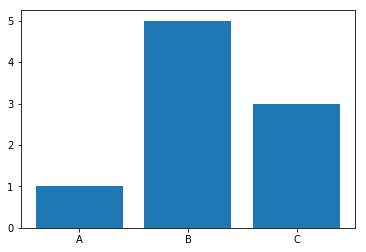Here, we’ve got a few categorical variables in a list – A, B and C. We’ve also got a couple of continuous variables in another list – 1, 5 and 3. The relationship between these two is then visualized in a Bar Plot by passing these two lists to plt.bar().

### Plot a Horizontal Bar Plot in Matplotlib¶

Oftentimes, we might want to plot a Bar Plot horizontally, instead of vertically. This is easily achieveable by switching the plt.bar() call with the plt.barh() call:

In :
import matplotlib.pyplot as plt

x = ['A', 'B', 'C']
y = [1, 5, 3]

plt.barh(x, y)
plt.show()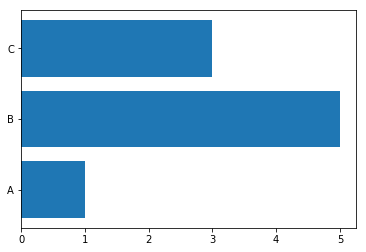### Change Bar Plot Color in Matplotlib¶

In :
import matplotlib.pyplot as plt

x = ['A', 'B', 'C']
y = [1, 5, 3]

plt.bar(x, y, color=['red', 'blue', 'green'])
plt.show()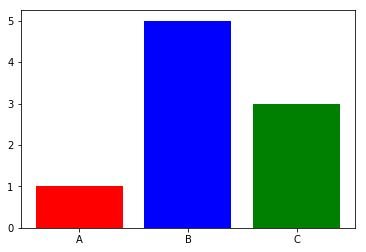Of course, you can also use the shorthand versions or even HTML codes:

In :
import matplotlib.pyplot as plt

x = ['A', 'B', 'C']
y = [1, 5, 3]

plt.bar(x, y, color=['r', 'b', 'g'])
plt.show()### or¶

In :
import matplotlib.pyplot as plt

x = ['A', 'B', 'C']
y = [1, 5, 3]

plt.bar(x, y, color=['#ff0000', '#00ff00', '#0000ff'])
plt.show()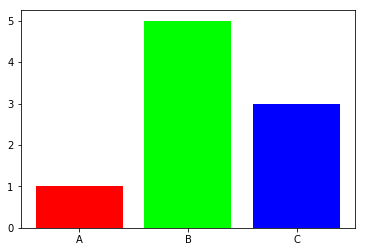Or you can even put a single scalar value, to apply it to all bars:

In :
import matplotlib.pyplot as plt

x = ['A', 'B', 'C']
y = [1, 5, 3]

plt.bar(x, y, color='green')
plt.show()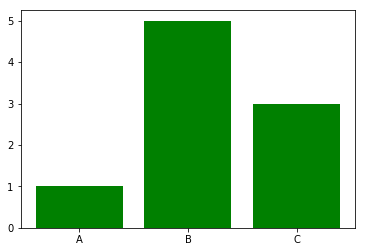### Change Width of BAR¶

In :
import matplotlib.pyplot as plt

plt.xlabel('x - axis')
plt.ylabel('y - axis')
plt.title('My bar chart!')

left = [1, 2, 3, 4, 5]
height = [10, 24, 36, 40, 5]

plt.bar(left, height, width = 0.8, color = ['red', 'green'])
plt.show()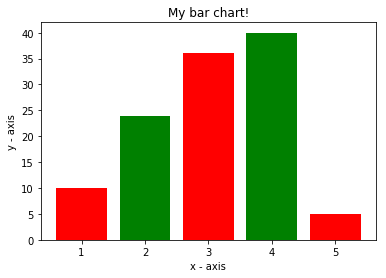### Categorical x-axis order – Bar Plot¶

In :
import matplotlib.pyplot as plt

plt.xlabel('x - axis')
plt.ylabel('y - axis')
plt.title('My bar chart!')

left = [1, 2, 3, 4, 5]
height = [10, 24, 36, 40, 5]

tick_label = ['one', 'two', 'three', 'four', 'five']

plt.bar(left, height, tick_label = tick_label, width = 0.8, color = ['red', 'green'])
plt.show()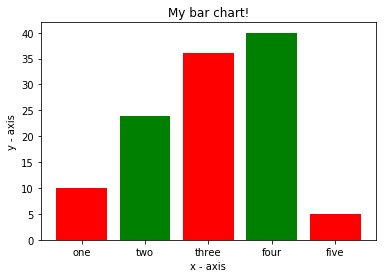### Multibar Plot¶

In :
x1=[2,3,6]
y1=[6,3,4]

x2=[3,5,7]
y2=[7,3,6]

plt.bar(x1,y1,width=0.5)
plt.bar(x2,y2,width=0.5)
plt.show()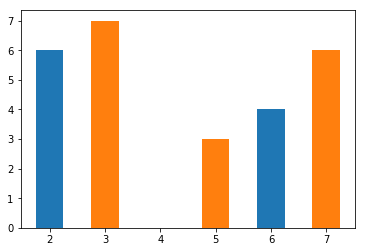In :
x1=[2,3,6]
y1=[6,3,4]

x2=[3,5,7]
y2=[7,3,6]

plt.bar(x1,y1,width=0.5)
plt.bar(x2,y2,width=0.5,alpha=.4) # alpha -> float (0.0 transparent through 1.0 opaque)
plt.show()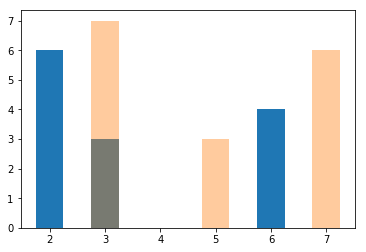In :
import numpy as np

N = 5
men = (20, 35, 30, 35, 27)
women = (25, 32, 34, 20, 25)

index = np.arange(N)
width = 0.35
plt.bar(index, men, width, label='Men')
plt.bar(index + width, women, width,label='Women')

plt.ylabel('Scores')
plt.title('Scores by group and gender')

plt.xticks(index + width / 2, ('G1', 'G2', 'G3', 'G4', 'G5'))
plt.legend(loc='best')
plt.show()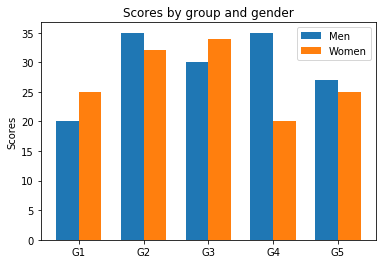### Bar Plot with Percentage¶

In :
import numpy as np
plt.ylabel('Scores')
plt.title('Scores by group and gender')

N = 5
men = (20, 35, 30, 35, 27)
women = (25, 32, 34, 20, 25)

index = np.arange(N)
width = 0.35

a = plt.bar(index, men, width, label='Men')
b = plt.bar(index + width, women, width,label='Women')

for rect in a:
height = rect.get_height()
plt.text(rect.get_x() + rect.get_width()/2., 1.04*height,'%.1f' % float(height),ha='center', va='bottom')

for rect in b:
height = rect.get_height()
plt.text(rect.get_x() + rect.get_width()/2., 1.04*height,'%.1f' % float(height),ha='center', va='bottom')

plt.ylim(0,45)

plt.xticks(index + width / 2, ('G1', 'G2', 'G3', 'G4', 'G5'))
plt.legend(loc='best')
plt.show()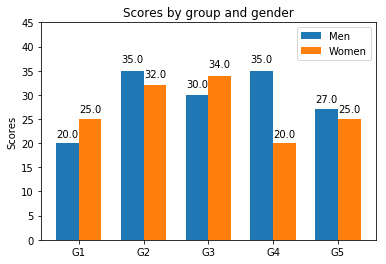### Tripple Bar Plot¶

In :
import numpy as np
import matplotlib.pyplot as plt

data = [[30, 25, 50, 20],[40, 23, 51, 17],[35, 22, 45, 19]]
X = np.arange(4)

plt.bar(X + 0.00, data, color = 'b', width = 0.25)
plt.bar(X + 0.25, data, color = 'g', width = 0.25)
plt.bar(X + 0.50, data, color = 'r', width = 0.25)

plt.show()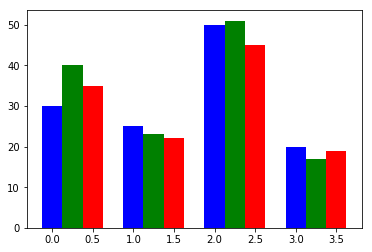### Matplotlib Style¶

In :
from matplotlib import style
print(plt.style.available)

['bmh', 'classic', 'dark_background', 'fast', 'fivethirtyeight', 'ggplot', 'grayscale', 'seaborn-bright', 'seaborn-colorblind', 'seaborn-dark-palette', 'seaborn-dark', 'seaborn-darkgrid', 'seaborn-deep', 'seaborn-muted', 'seaborn-notebook', 'seaborn-paper', 'seaborn-pastel', 'seaborn-poster', 'seaborn-talk', 'seaborn-ticks', 'seaborn-white', 'seaborn-whitegrid', 'seaborn', 'Solarize_Light2', 'tableau-colorblind10', '_classic_test']

In :
import matplotlib.pyplot as plt
from matplotlib import style
import numpy as np
style.use('ggplot')

plt.ylabel('Scores')
plt.title('Scores by group and gender')

N = 5
men = (20, 35, 30, 35, 27)
women = (25, 32, 34, 20, 25)

index = np.arange(N)
width = 0.35

a = plt.bar(index, men, width, label='Men')
b = plt.bar(index + width, women, width,label='Women')

for rect in a:
height = rect.get_height()
plt.text(rect.get_x() + rect.get_width()/2., 1.04*height,'%.1f' % float(height),ha='center', va='bottom')

for rect in b:
height = rect.get_height()
plt.text(rect.get_x() + rect.get_width()/2., 1.04*height,'%.1f' % float(height),ha='center', va='bottom')

plt.ylim(0,45)

plt.xticks(index + width / 2, ('G1', 'G2', 'G3', 'G4', 'G5'))
plt.legend(loc='best')
plt.show()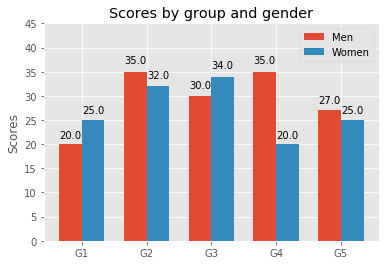In [ ]: